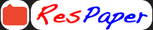Trending ▼   ResFinder# CBSE Pre Board Class 10 2021 : Mathematics - Prelim 1 (Matushri Kashiben Vrajlal Valia International Vidyalaya (MKVV), Borivli, Mumbai)

7 pages, 62 questions, 0 questions with responses, 0 total responses,20Ashok Badlani  Mumbai +Fave Message ProfileTimelineUploads
 Home > ashok6644 >   F Also featured on: School Page cbse10_pre_boards and 1 moreFormatting page ...

MKVV INTERNATIONAL VIDYALAYA STD X ACADEMIC YEAR 2020 21 SUB MATHS PRELIMS MARKS 80 TIME 3hrs General instructions: 1. This question paper contains two parts A and B 2. Both Part A and Part B have internal choices Part- A 1. It consists of sections I and II 2. Section I has 16 questions of 1 mark each . 3. Section II has 4 questions on case study .Each case study has 5 case based sub parts. Attempt any 4 out of 5 sub parts PART - B 1. Question No 21 to 26 carry of 2 marks each 2. Question No 27 to 33 carry 3 marks each 3 Question No 34 to 36 carry 5 marks each PART A Section - 1 Section 1 has 16 questions of 1 mark each. 1. On comparing the ratios write weather the following pair of equation is consistent or not 3x + 4y +5 = 0 , 6x + 8y + 10 = 0 2. Two dice are thrown simultaneously .Find the probability of getting a sum 7 3 . Find the area of a quadrant of a circle where the circumference is 44cm OR If the diameter of a semi circular protractor is 14cm , then find its perimeter 4. Find the value of x if tan3x = sin 45 cos45 + sin30 5. If and are the zeros of the polynomial x - 5x + k , where = 1 , find the value of k 6 . If 2p + 1 , 13, 5p 3 are three consecutive terms of an A.P , then find the value of p OR th The 17 term of an A.P. exceeds its 10th term by 7. Find the common difference 7. Draw a line segment of length 8cm .Divide it internally in the ratio 3: 4 8. For what value of k, the following pairs of equations has no solutions 2x + 3y = 5 , 6x + ky = 15 9. Solve for x : 9x - 3( a + b) x + ab = 0

Related ResPapers
 CBSE Class 10 Pre Board 2021 : Mathematics (Kendriya Vidyalaya (KV) No 1, Armapur, Kanpur) by cbse10_pre_boards CBSE Class 10 Pre Board 2021 : Mathematics (Toc H Public School, Kochi) by macandcheese73 CBSE Class 10 Pre Board 2021 : Mathematics Standard - Set 4 (CBSE Gulf Sahodaya Qatar Chapter, Doha) by cbse10_pre_boards CBSE Class 10 Pre Board 2020 : Mathematics Standard - Prelim 1 (Kendriya Vidyalaya (KV) Agra) by cbse10_pre_boardsFormatting page ...Formatting page ...Formatting page ...Formatting page ...Formatting page ...Formatting page ...